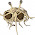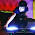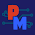Tuesday, July 17, 2012

How Does a Power Supply regulate It’s Output Voltage and Current?

We have talked about Constant Voltage (CV) and Constant Current (CC) power supply operation in many various ways and applications here on the “Watt’s Up?” blog in the past. Indeed, CV and CC are fundamental operating modes of most all power supplies. But what exactly takes place inside the power supply that endows it with the ability to regulate either its output voltage or current, depending on the load? If you ever wondered about this, wonder no longer!

Most all power supplies regulate either their output voltage or output current at a constant level, depending on the load resistance relative to the power supply’s output voltage and current settings. This can be summarized as follows:

·         If R load > (V out / I out) then power supply is in CV mode
·         If R load < (V out / I out) then power supply is in CC mode

To accomplish this most all power supplies have separate voltage and current feedback control loops to limit either the output voltage or current, depending on the load. To illustrate this Figure 1 shows a circuit diagram of a basic 5 volt, 1 amp output series regulated power supply operating in CV mode.
Figure 1: Basic DC Power Supply Circuit, Constant Voltage (CV) Operation

The CV and CC control loops/amplifiers each have a reference input value. In this case the reference values are both 1 volt. In order to regulate output voltage the CV error amplifier compares its 1 volt reference against a resistor divider that divides the output voltage down by a factor of 5, limiting the output voltage to 5 volts. Likewise the CC error amplifier compares its 1 volt reference against a 1 ohm current shunt resistor located in the output current path, limiting the output current to 1 amp. For Figure 1 the load resistance is 10 ohms. Because this load resistance is greater than (V out / I out) = 5 ohms, the power supply is operating in CV mode. The CV error amplifier takes control of the series pass transistor by drawing away excess base current from the series pass transistor, though the diode “OR” network. The CV amplifier is operating in closed loop, maintaining its error voltage at zero volts. In comparison, because the actual output current is only 0.5 amps the CC amplifier tries to turn the current on harder but cannot because the CV amplifier has control of the output. The CC amplifier is operating open loop. Its output goes up to its positive limit while it has -0.5 volts of error voltage. The output I-V diagram for this Constant Voltage operation is shown in Figure 2.
Figure 2: Power Supply I-V Diagram, CV Operation

Now say we increase the load by lowering the output load resistance from 10 ohms down to 3 ohms. Figure 3 shows the circuit diagram of our basic 5 volt, 1 amp output series regulated power supply revised for operating in CC mode with a 3 ohm load resistor.
Figure 3: Basic DC Power Supply Circuit, Constant Current (CC) Operation

Because the load resistor is lower than (V out / I out) = 5 ohms, the power supply switches to CC mode. The CC error amplifier takes control when the voltage drop on the current shunt resistor increases to match the 1 volt reference value, corresponding to 1 amp output, drawing excess base current from the series pass transistor though the diode “OR” network. The CC amplifier is now operating closed loop, regulating the output current to maintain its input error voltage at zero. In comparison, because the actual output voltage is now only 3 volts the CV amplifier tries to increase the output voltage but cannot because the CC amplifier has control of the output. The CV amplifier is operating open loop. Its output now goes up to its positive limit while it has -0.4 volts of error voltage. The output I-V diagram for this Constant Current operation is shown in Figure 4.
Figure 4: Power Supply I-V Diagram, CC Operation

As we have seen most all power supplies have separate current and voltage control loops to regulate their outputs in either a Constant Voltage (CV) or in a Constant Current (CC) mode. One or the other takes control, depending on that the load resistance is in relation to what the power supply’s output voltage and current settings are. In this way both the load and power supply are protected by limiting the voltage and current that is delivered by the power supply to the load. By understanding this theory behind a power supply’s CV and CC operation it is also easier to understand the underlying reason for why various power supply characteristics are the way they are, as well as see how other power supply capabilities can be created by building on top of this foundation. Stay tuned!

1.How do you calculate the "R pull up"?

1.May (VCC - VB) / ib, since ib = beta x ic

2.Hi, the circuit shown is mainly intended to conceptually show the principles of how feedback provides control of the output. In a practical design the pull up resistor would be selected to furnish sufficient base current to the power transistor for it to drive its full output current, based on the current gain of the transistor.

3.I saw some power supply circuit with the output regulable for example from 0 to 30 Volt and the op amp supply with +/- 5 Volt.
I dont understand how the max output voltage can be highest of the voltage supply of the op amp.
In a simple circuit like this: http://imageshack.us/scaled/large/706/4iaw.png, the output voltage does not exceed the supply voltage of the op amp although on the collector of the transistor there are 35 Volt.

4.I looked at the circuit you referenced and I agree it would not work as shown. It would need another stage placed between the output of the op amp and the input of the power pass transistor to provide a level shifting between the op amp and transistor.

5.For example?

6.For example in this circuit:

http://imageshack.us/scaled/large/689/o7rz.jpg

The output voltage is regulable from 0 to 15 or 30 Volt but the OP are supply with +/- 5 Volt ...
The transistor T1, seems a costant current generator for the bases of T2 and T3 ... and i noticed that the 0 volt of the OP supply stage is connected with the positive output of the main power supply.
I don't know if these things are the key.

1.Hi MangustaCBT,
I looked at the last circuit and the main key is the op amps are biased from the floating power suppy that referenced to V+. This way they do not see the large difference of voltage that exists at the output. This is pretty common in a practical design with higher voltage. From your previous question regarding putting in a level shifter a simple approach would be to use a common emitter NPN transistor, where the base side is driven by the op amp and then the collector side, at higher voltage, can pull down on the base of the pass transistor. A pull-up resistor between the base and collector of the pass transistor would be needed. This would also introduce a phase reversal which would need to be offset by reversing the inputs on the op amp, for example.

7.This comment has been removed by the author.

8.how to bias op amps......

9.how to bias op amps......

10.how to bias op amps......

1.Hi,
The circuit shown is mainly meant to be a conceptual diagram to show the feedback mechanisms controlling the output. However for most op amps their input and output voltages need to be in between their "+" and "-" bias voltage rails. For the circuit shown, using "+" and "-" 15 volts, referenced to the "-" of the main power voltage, would more than adequately meet this need.

11.I dont think this is the concept of current limiting, what you have here is two voltage limiters with different references, a constant current would be if u still mantain the output potential, lets say if u have 5v and 1A on a load, you want to limit at 200mA, the circuit should limit at 200mA but still maintain the 5V output on the same load. this is where the FET comes in, because narrowing his channel limits only the current mentaining the same output potential voltage, so instead of the diode OR, the current amp should control a series FET before or after the voltage regulator series transistor.

1.Hi Quest, Those are very interesting thoughts. But if you have 5 volts and 1 amp on the load, the load would have to be 5 ohms. If you then change the current limit to 200 mA you would not be able to maintain 5V output on the same load as the voltage would have to drop to 1 volt, given its a 5 ohm load. The characteristic of the two limiter circuits depend on what type of signal they are monitoring for feedback control. In this case one is monitoring output voltage and so its a voltage limiter. The second one is monitoring the output current, so its a current limiter. While it might be feasible to use two power control elements, one for current and one for voltage, it is far more common and customary to just use one as depicted, in a power supply design

12.Hi! I have a related question (googleing it I drop myself there!) I have a 12V 13A (156W) heater. Basically a fan behind a 150W resistor. My 12V socket car where I plug and use has a 10A fuse (that i don't want to change in order protect other things) so the power output disintegrate the fuse.
I just want to know which is the cheap common piece of electronic I could install inside the heater that could limitate the current output from 13A to 9A for example.
Thank you very much with this amazing knowledgeable site and sorry about the off-topic question!

1.I mean a piece of electronic to limit the current input inside the heater (current consumption) in order to only require 9A of max current through the 12V socket. So I don't modify any other thing than the heater.

2.This comment has been removed by the author.

13.An inexpensive and common electronic part you can install in your heater would be a power resistor. This will work because your heater is basically a fixed resistor as well. Adding an 0.45 ohm 33 watt resistor in series with the heater element will reduce the current of the heater to 9 amps. Of course you want the fan blowing the heat off the resistor as well!

14.perfectly understood, Thank You Ed!

15.can we do the design with using mosfet to reduce the base drive current and there will be any advantage for the same for making 0 to 60V 50 amps dc load jig.

16.The circuit is meant mainly to illustrate the feedback control mechanism, but yes, a MOSFET could be used in place of the BJT to minimize/eliminate drive current. This would be true whether the circuit is for a power supply or an electronic load.

17.I have for a while been trying to learn more about power supply design, and have already built basic buck converter based on the popular LM2596 and some crude linear reg's using just a Sziklai pair and LED as a crude voltage reference! Worked kinda well really, it had really solid load regulation, but the line regulation was a bit iffy.

I have recently aquired a few really nice (and really heavy!!!) LAMBDA power supplies, however one of them didn't work at all, so I hacked it and bypassed the regulation circuitry. It had a nominal rating of 5V 10.8A, but now has an unregulated output of 17V with center tap rectification. My larger regulated 24V 9A one does work, but has annoying foldback current limiting, which causes the output to go to crap when even a short duration overload is detected.

I would like to modify this power supply to give me (ideally) completly variable 0-15v dual rail voltages and 0-9A adjustable current limit, and I would like this to be controlled by a 16 bit DAC from an arduino. (I have a pile of REALLY old DAC71 chips) One of my cool visions with this idea is that I can use a nice LCD display and control the supply remotely with a bluetooth or wifi app, and possibly do data logging which could come in handy for efficiency measurements and stuff!

However, I think I might run into issues regarding the power dissipation at low output voltages and high currents, and that I have not been able to make a proper voltage and current limited power supply work without parasitic operation under certain conditions on a breadboard or LTspice.

I would like this mod to be made mostly from the spare parts I have, which include literally HANDFULS of LM741's, LM324's, and other LT brand op amps, about 10 TO-3 style 2N3055Ts and IRFP250 MOSFETs, and lots of TTL 7400 series logic!

Do you have any tips on where I can learn more about the design of linear regulators? Or any tips on creating a simple but robust design that will handle all the abuse I can realistically give it? (like inductive loads and stuff!)

1.I just built (a slightly more sophisticated and practical) version of this circuit, incorporating the error amplifier design for the current limiting, and it worked almost flawlessly first try!!! My old solution used 4 op amps, 1 for the voltage error amp, one for use for a CV or CC LED driver, one as a differential amplifier for the shunt resistor, and one as a comparator directly following that. That way my the current "reference" is an absolute voltage above ground, which would so happen to be the DAC like I mentioned. However I think the solution here is a LOTTT better since that "hard" 2 op-amp solution was very prone to parasitic oscillation.

2.Sorry for all these long comments, I forgot to add that I only built it so far in a LTspice simulator using 2 LT1014A's, ideal resistors, ideal voltage sources, and I have not thoroughly tested the circuit yet for line and load regulation.

18.How to make continuous adjustable current dc power supply

1.Hi, referring to Figure 3, if the Iout reference is made continuously adjustable, then you would have a continuously adjustable output current

Note: Only a member of this blog may post a comment.